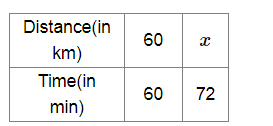# Mark (✓) against the correct answer:

Question:

Mark (✓) against the correct answer:

A car is travelling at an average speed of 60 km per hour. How much distance will it cover in 1 hour 12 minutes?

(a) 50 km

(b) 72 km

(c) 63 km

(d) 67.2 km

Solution:

(b) 72 km

Let x km be the distance covered in 1 h 12 min.

Now, 1 h 12 min = (60+12) min = 72 minMore distance will be covered in more time.

So, it is a cas of direct proportion.

Now, $\frac{60}{60}=\frac{x}{72}$

$\Rightarrow x=72 \mathrm{~km}$

$\therefore$ The car will cover a distance of $72 \mathrm{~km}$ in $1 \mathrm{~h} 12 \mathrm{~min}$.